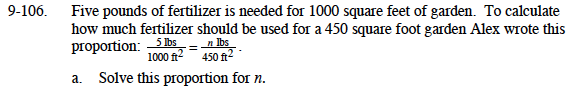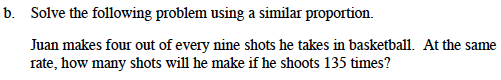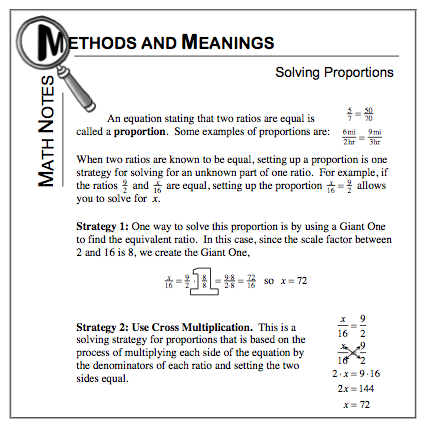### Home > MC1 > Chapter 9 > Lesson 9.2.5 > Problem9-106

9-106.To solve this proportion, it would be useful to use cross multiplication. With this strategy you should multiply each side of the equation above by the denominators of each fraction. Then set the sides equal to each other and solve for n.

Using cross multiplication, your expression should look something like this: 5 lbs. (450 ft2) = 1000 ft2 (n lbs.)
Can you multiply the left side and then find the value of n?$\text{If Juan makes four of nine shots, this is a ratio of }\frac{4}{9}.$

Your goal is to change this ratio to a ratio over 135. Can you make a proportion now?

$\text{Your proportion might look something like this: }\frac{4}{9}=\frac{x}{135}.$

Can you solve the proportion? Refer to the Math Notes box below if you are having trouble.

Juan will make 60 shots if he shoots 135 times. Did you remember to show your work?